IdentifiantMot de passe
Mot de passe oublié ?

Inscrivez-vous gratuitement
pour pouvoir participer, suivre les réponses en temps réel, voter pour les messages, poser vos propres questions et recevoir la newsletter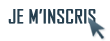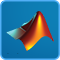Discussion :

# Erreur script matlab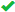Sujet :

## MATLAB

1.Erreur script matlab
Salut a tous et merci pour lire ma question!

J'ai fait un script en matlab qui utilise le script load_image.m qui a le contenu suivant:

 Code : Sélectionner tout - Visualiser dans une fenêtre à part
```12345678910111213141516171819202122232425262728293031323334353637383940414243444546474849505152535455565758596061626364656667686970717273747576777879808182838485868788899091

%
%
%   type can be
%   'chessboard1', 'boat', 'lena', 'goldhill', 'mandrill', 'maurice'.
%
%   Copyright (c) 2004 Gabriel Peyr

if nargin<2
n = 512;
end

type = lower(type);

%%%%%%%%%%%%%%%%%%%%%%%%%%%%%%%%%%%%%%%%%%%%%%%%%%%%%%%%%%%%%%%%%%%%%%%%%
% for the line, can be vertical / horizontal / diagonal / qcq
if strcmp(type, 'line')
eta = 0.1;              % translation
gamma = 1/sqrt(2);      % slope
elseif strcmp(type, 'line_vertical')
eta = 0.5;              % translation
gamma = 0;      % slope
elseif strcmp(type, 'line_horizontal')
eta = 0.5;              % translation
gamma = Inf;      % slope
elseif strcmp(type, 'line_diagonal')
eta = 0;              % translation
gamma = 1;      % slope
end

%%%%%%%%%%%%%%%%%%%%%%%%%%%%%%%%%%%%%%%%%%%%%%%%%%%%%%%%%%%%%%%%%%%%%%%%%
switch type
case 'chessboard1'
x = -1:2/(n-1):1;
[Y,X] = meshgrid(x,x);
y = (2*(Y>=0)-1).*(2*(X>=0)-1);

case 'square'
x = -1:2/(n-1):1;
[Y,X] = meshgrid(x,x);
y = max( abs(X),abs(Y) )<r;

case {'line', 'line_vertical', 'line_horizontal', 'line_diagonal'}
x = 0:1/(n-1):1;
X = x'*ones(1,n);
if gamma~=Inf
y = (X-eta) - gamma*X' < 0;
else
y = (X'-eta) < 0;
end

case 'disk'
x = 0:1/(n-1):1;
[Y,X] = meshgrid(x,x);
center = [0.5, 0.5];    % center of the circle
y = (X-center(1)).^2 + (Y-center(2)).^2 < radius^2;

case 'quarterdisk'
x = 0:1/(n-1):1;
[Y,X] = meshgrid(x,x);
center = -[0.1, 0.1];    % center of the circle
y = (X-center(1)).^2 + (Y-center(2)).^2 < radius^2;

case '3contours'
r1 = 1.3;
ct1 = [-1, 1];
r2 = 0.8;
ct2 = [0, 0];
x = 0:1/(n-1):1;
[Y,X] = meshgrid(x,x);
f1 = (X-ct1(1)).^2 + (Y-ct1(2)).^2 < r1^2;
f2 = (X-ct2(1)).^2 + (Y-ct2(2)).^2 < r2^2;
y = f1 + 0.5*f2.*(1-f1);

otherwise
y = imread( [type, '.jpg'] );
y = double(y);
if n~=size(y, 1)
y = image_resize(y,n,n);
end
end

y = double(y);```
Le probleme c'est que mon script n'a pas aucune probleme mais malheuresement il se lie avec load_image.m et il me donne a cette ligne:

 Code : Sélectionner tout - Visualiser dans une fenêtre à part
`y = image_resize(y,n,n);`
L'erreur suivante:

 Code : Sélectionner tout - Visualiser dans une fenêtre à part
```1234??? Undefined function or method 'image_resize' for input arguments of type 'double'.

Error in ==> load_image at 86
y = image_resize(y,n,n);```
Si quelqu'un peut m'aider comment faire pour supprimer l'erreur je serais reconnaisant!

Parce que ca me bloque pour avancer dans mon projet.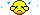Répondre avec citation   0  0

2.Bonjour,

ou as-tu récupéré le script load_image.m? Il n'y avait pas un script image_resize.m avec?Répondre avec citation   0  0

3.Pour être précis, ce ne sont pas des scripts mais des fonctions... la différence se situe dans la déclaration de la première ligne du fichier mRépondre avec citation   0  0

4.Oui, effectivement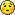ce qui est sur, c'est qu'il manque un fichier pour que MATLAB puisse continuer....Répondre avec citation   0  0

5.Oui c'etait ca.

Maintenant ca me donne une autre erreur dans mon script.

Je vous explique en fait ce que je suis en train de faire. Je veux faire la transformation haar 2d en utilisant le paragraphe "Forward 2D Haar transform"

J'ai déjà les scripts load_image.m et image_resize dans le path du matlab.

Voila le code: (haar_transf.m)

 Code : Sélectionner tout - Visualiser dans une fenêtre à part
```12345678910111213141516171819202122232425262728293031323334353637383940414243444546474849505152535455565758596061626364656667
% haar 2d transform

% imshow(RGB);
% title('This is the 102.jpg picture in RGB mode');

%  % read the grayscale image

%  I  = rgb2gray(RGB); % convert to grayscale
%  figure,imshow(I);
%  title('This is the 102.jpg picture in GRAYSCALE mode');

name = 'photo_grey';
n = 256;

%M = rescale(crop(M,n));

MW = M;
j = log2(n)-1;

%Select the sub-part of the image to transform.

A = MW(1:2^(j+1),1:2^(j+1));

%Compute average and differences along the vertical direction.

Average_vert = ( A(1:2:size(A,1),:) + A(2:2:size(A,1),:) )/sqrt(2);
Differences_vert = ( A(1:2:size(A,1),:) - A(2:2:size(A,1),:) )/sqrt(2);

%Concatenate them in the vertical direction to get the result.

A = cat3(1, Average_vert, Differences_vert );

% Display the result of the vertical transform.

%clf; % clear figure
imageplot(M,'Original image',1,2,1);
imageplot(A,'Vertical transform',1,2,2);

% Compute average and differences along the horizontal direction.

Average_horiz = ( A(:,1:2:size(A,1)) + A(:,2:2:size(A,1)) )/sqrt(2);
Differences_horiz = ( A(:,1:2:size(A,1)) - A(:,2:2:size(A,1)) )/sqrt(2);

% Concatenate them in the horizontal direction to get the result.

A = cat3(2, Average_horiz, Differences_horiz );

% Assign the transformed data.

MW(1:2^(j+1),1:2^(j+1)) = A;

% Display the result of the horizontal transform.

clf;
imageplot(M,'Original image',1,2,1);
subplot(1,2,2);
plot_wavelet(MW,log2(n)-1);
title('Transformed');```
Quand je lance haar_transf.m

 Code : Sélectionner tout - Visualiser dans une fenêtre à part
`>>haar_transf`
Erreur:

 Code : Sélectionner tout - Visualiser dans une fenêtre à part
```12345
??? Undefined function or method 'cat3' for input arguments of type 'double'.

Error in ==> haar_transf at 39
A = cat3(1, Average_vert, Differences_vert );```
Il est possible que cat3 est aussi une fonction mais j'ai pas trouve le script.

La meme chose pour plot_wavelet et imageplot.

Merci de m'aider!

Voila la photo nomme photo_grey parce seulement a ca je peux faire la transformation wavelet. J'ai encore des soucis mais pour l'instant je veux resoudre ca: (puis j'ouvre un autre message avec l'autre souci que j'ai)

http://img13.imageshack.us/img13/7281/photogrey.jpg

J'attends vos avis!

Amicalment,

MichelRépondre avec citation   0  0

6.Je pense que c'est une erreur de typo :
 Code : Sélectionner tout - Visualiser dans une fenêtre à part
```12
A = cat3(2, Average_horiz, Differences_horiz );```
devient simplement
 Code : Sélectionner tout - Visualiser dans une fenêtre à part
```12
A = cat(2, Average_horiz, Differences_horiz );```Répondre avec citation   0  0

7.J'ai resolu!

Merci beaucoup a tous!

Maintenant ce que je veux c'est trouver une maniere pour stoquer pour une image les coefficient wavelet qui decrit le degre de blur d'une image.

Donc j'ouvre un autre poste qui se penche sur "haar 2d approximation".

Si quelqu'un a besoin de la source il peut la trouver ici.

http://www.mediafire.com/?t2gondowgjvRépondre avec citation   0  0

+ Répondre à la discussion
Cette discussion est résolue.
 Actualités FAQs MATLAB Tutoriels MATLAB Livres MATLAB Sources MATLAB